# Ray-Casting & Ray-Tracing with VTK

January 30, 2015VTK has long evolved beyond just visualization. It offers some amazing functionality that just cannot be found elsewhere. Two examples are the ‘ray-casting’ and, consequentially, ‘ray-tracing’ capabilities provided by the vtkOBBTree class. In this article, I would like to introduce these capabilities and show examples of ray-casting and ray-tracing performed exclusively through Python, a dash of NumPy, and VTK.

Disclaimer: The ray-casting and ray-tracing examples I will be presenting here are severely condensed versions of my posts “Ray Casting with Python and VTK: Intersecting lines/rays with surface meshes” and “From Ray Casting to Ray Tracing with Python and VTK” that appear on my blog . If they pique your interest, please visit the aforementioned posts, where you can find all of the material and code (in the form of IPython Notebooks), as well as an excruciating amount of detail pertaining to each aspect of the process, as these posts were written for people with little to no experience in VTK.

Ray-Casting vs. Ray-Tracing

I would like to emphasize a pivotal difference between ‘ray-casting’ and ‘ray-tracing.’ In the case of the former, we only ‘cast’ a single ray, test for its intersection with objects, and retrieve information regarding the intersection. Ray-tracing, on the other hand, is more physically accurate, as it applies laws of physics (e.g., reflection, refraction, attenuation, etc.) to the rays to ‘trace’ (i.e., follow) that ray and its derivative rays. However, ray-casting is the natural precursor to ray-tracing, as it tells us with what part of which object the ray intersects and provides all necessary information to cast subsequent rays.

The vtkOBBTree Class

The star of this post is the vtkOBBTree class, which generates an oriented bounding-box (OBB) ‘tree’ for a given geometry under a vtkPolyData object. Upon generation of this OBB tree, the vtkOBBTree class allows us to perform intersection tests between the mesh and the lines of finite length, as well as intersection tests between different meshes. It can then return the point coordinates where intersections were detected, as well as the polydata cell IDs where the intersections occurred.

Ray-Casting with vtkOBBTree

For this demonstration, we are assuming that we have a surface model of a human skull stored in a .stl file, whose contents we have loaded into a vtkPolyData object, named mesh, through the vtkSTLReader class. A rendering of this model through the vtkPolyDataMapper class can be seen in Figure 1.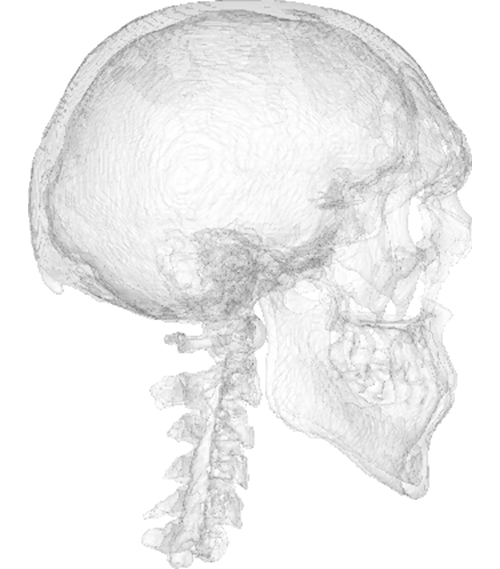Figure 1. Rendering of the surface model of a human skull, which we will use to demonstrate ray-casting.

The center of the skull is centered around the cartesian origin, i.e., the (0.0, 0.0, 0.0) point. Now let’s assume we want to cast a ray emanating from (100.0, 100.0, 0.0) and ending at (0.0, 0.0, 0.0) and retrieve the coordinates of the points where this ray intersects with the skull’s surface. A rendering including the ray, prior to actually casting it, can be seen in Figure 2.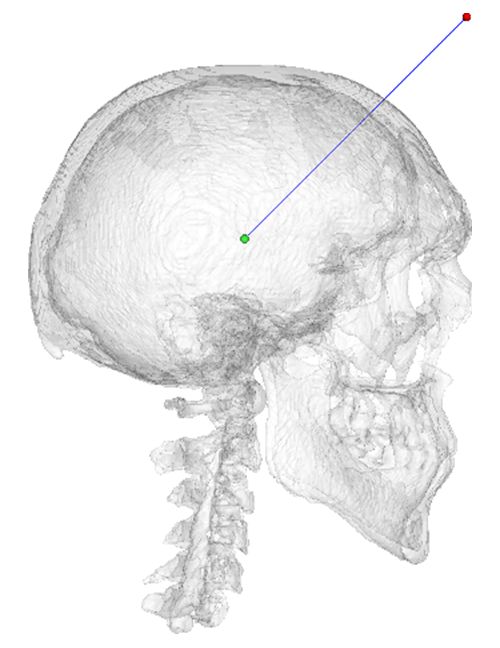Figure 2. The ray that will be tested for intersection with the skull model. The ‘source’ point of the ray is rendered as red, while the ‘target’ point is rendered as green.

Prior to intersection, we need to create and initialize a new vtkOBBTree with the vtkPolyData object of our choice. In our case, this is called mesh and is done as follows:

```obbTree = vtk.vtkOBBTree()
obbTree.SetDataSet(mesh)
obbTree.BuildLocator()```

Note the call to the BuildLocator method, which creates the OBB tree.

That’s it! We now have a world-class intersection tester at our disposal. At this point, we can use the
IntersectWithLine method of the vtkOBBTree class to test for intersection with the aforementioned ray. We merely need to create a vtkPoints object and a vtkIdList object to store the results of the intersection test.

```points = vtk.vtkPoints()
cellIds = vtk.vtkIdList()

code = obbTree.IntersectWithLine((100.0, 100.0, 0.0), (0.0, 0.0, 0.0), points, cellIds)```

As mentioned above, the points and cellIds now contain the point coordinates and cell IDs with which the ray intersected as it was emanated from the first point, i.e., (100.0, 100.0, 0.0), onto the second point, i.e., (0.0, 0.0, 0.0), in the order they were ‘encountered.’ The return value code is an integer, which would be equal to 0 if no intersections were found. A rendering showing the intersection points can be seen in Figure 3.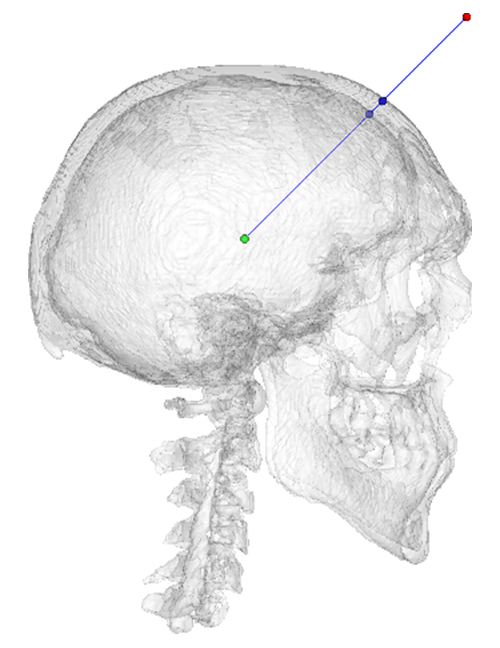Figure 3. Result of the ray-casting operation and intersection test between the skull model and the ray. The blue points depict the detected intersection points.

The Python package pycaster  wraps the functionality shown above, assuming no VTK experience, and provides additional methods to calculate the distance a given ray has ‘traveled’ within a closed surface. It is currently being served through PyPI. The repository  can be found on BitBucket.

As I mentioned at the beginning of this article, the entire process shown above, including all of the material and code needed to reproduce it, is detailed in my post “Ray Casting with Python and VTK: Intersecting lines/rays with surface meshes” .

Ray-Tracing with vtkOBBTree

Now, in order to perform ray-tracing, we can take the lessons learned from ray-casting and apply them to a more convoluted scenario. The rationale behind ray-tracing with the vtkOBBTree class is the following:

• Cast rays from every ‘ray source’ and test for their intersection with every ‘target’ mesh in the scene.
• If a given ray intersects with a given ‘target,’ then use the intersection points and intersected cells to calculate the normal at that cell, to calculate the vectors of the reflected/refracted rays, and to cast subsequent rays off the target.
• An excellent, freely available article entitled “Reflections and Refractions in Ray-Tracing”  (Bram de Greve, 2006)  provides a good overview of the math and the physics behind ray-tracing.
• Repeat this process for every ray cast from the ‘ray source.’

Let’s assume a scene is comprised of a half-sphere dubbed sun, which will act as the ray-source, and a larger nicely textured sphere called earth, which will be the target of those rays. This ‘environment’ can be seen in Figure 4.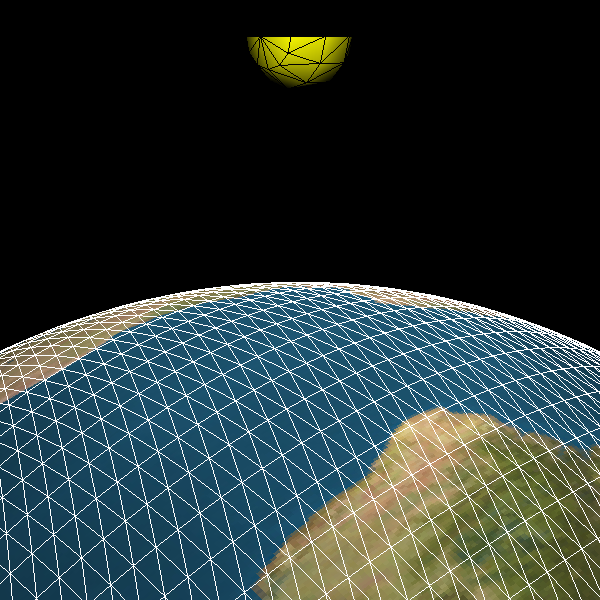Figure 4. Scene defined for the ray-tracing example. The yellow half-sphere, sun, acts as the ray-source, while the textured sphere, earth, will be the target of those rays.

In this example, we will be casting a ray from the center of each triangular cell on the sun’s surface along the direction of that cell’s normal vector. The cell-centers of the sun were calculated through the vtkCellCenters class and stored under pointsCellCentersSun (of type vtkPolyData). The cell-normals of the sun were calculated through the vtkPolyDataNormals class and stored under normalsSun (of type vtkFloatArray). A rendering of the cell-centers as points and cell-normals as glyphs through the vtkGlyph3D class can be seen in Figure 5.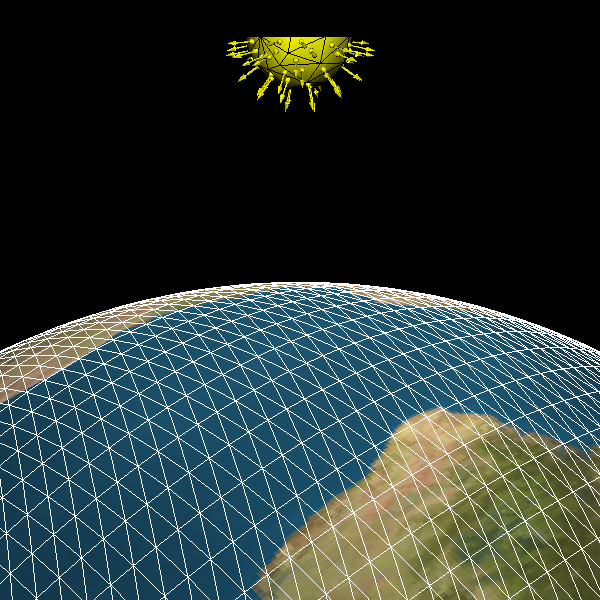Figure 5. Rendering of the cell-centers of the sun that will act as source-points for the rays and the cell-normals along which the rays will be cast.

Similarly to what was done in the previous example, prior to ray-casting, we first need to create a vtkOBBTree object for the earth:

```obbEarth = vtk.vtkOBBTree()
obbEarth.SetDataSet(earth.GetOutput())
obbEarth.BuildLocator()```

Now, since we will be casting a large number of rays, let’s wrap the vtkOBBTree functionality in two convenient
functions:

```def isHit(obbTree, pSource, pTarget):
code = obbTree.IntersectWithLine(pSource, pTarget, None, None)
if code==0:
return False
return True

def GetIntersect(obbTree, pSource, pTarget):
points = vtk.vtkPoints()
cellIds = vtk.vtkIdList()
# Perform intersection test
code = obbTree.IntersectWithLine(pSource, pTarget, points, cellIds)

pointData = points.GetData()
noPoints = pointData.GetNumberOfTuples()
noIds = cellIds.GetNumberOfIds()

pointsInter = []
cellIdsInter = []
for idx in range(noPoints):
pointsInter.append(pointData.GetTuple3(idx))
cellIdsInter.append(cellIds.GetId(idx))

return pointsInter, cellIdsInter```

The isHit function will return True or False, depending on whether a given ray intersects with obbTree, which, in our case, will only be obbEarth.

The GetIntersect function simply wraps the functionality we saw in the first example. In a nutshell, it will return two list objects: pointsInter and cellIdsInter. The former will contain a series of tuple objects with the coordinates of the intersection points. The latter will contain the ‘id’ of the mesh cells that were ‘hit’ by that ray. This information is vital, as we will be able to get the correct normal vector for that earth cell and calculate the appropriate reflected vector through these ids, which we will see below.

At this point, we are ready to perform the ray-tracing. Let’s take a look at a condensed version of the code:

```noPoints = pointsCellCentersSun.GetNumberOfPoints()
for idx in range(noPoints):
normalSun = normalsSun.GetTuple(idx)

# Calculate the 'target' of the 'sun' ray based
# on 'RayCastLength'
pointRayTarget = list(numpy.array(pointSun) + RayCastLength*numpy.array(normalSun))

if isHit(obbEarth, pointSun, pointRayTarget):
pointsInter, cellIdsInter = GetIntersect(obbEarth, pointSun, pointRayTarget)

# Get the normal vector at the earth cell
# that intersected with the ray
normalEarth = normalsEarth.GetTuple(cellIdsInter)

# Calculate the incident ray vector
vecInc = (numpy.array(pointRayTarget) - numpy.array(pointSun))
# Calculate the reflected ray vector
vecRef = (vecInc - 2*numpy.dot(vecInc, numpy.array(normalEarth)) * numpy.array(normalEarth))

# Calculate the 'target' of the reflected
ray based on 'RayCastLength'
pointRayReflectedTarget = (numpy.array(pointsInter) + RayCastLength*l2n(vecRef))```

Please note that all rendering code was removed from the above snippet. What is done above is the following:

We looped through every cell-center on the sun mesh (pointSun), stored under pointsCellCentersSun.

We casted a ray along the direction of that sun cell’s normal vector, stored under normalsSun. The ray emanates from pointSun to pointRayTarget.

As the vtkOBBTree class only allows for intersection tests with lines of finite length, not semi-infinite rays, the rays cast in the code above are given a large (relative to the scene) length to ensure that failure to intersect with earth would only be due to the ray’s direction and not an insufficient length.

Every ray was tested for intersection with the earth through the isHit function and the obbEarth object defined above.

If a ray intersected with the earth, the intersection test was repeated through the GetIntersect function in order to retrieve the intersection point coordinates and the intersected cell IDs on the earth mesh. The intersection point coordinates and the intersected earth cell normal vector (normalEarth) were used to calculate the normal vector of the reflected ray and cast that ray off the earth’s surface.

The cell normals on the earth’s surface were calculated through the vtkPolyDataNormals class and stored under
normalsEarth (of type vtkFloatArray). This is the same way that normalSun was calculated.

A render of the ray-tracing result can be seen in Figure 6.

As I mentioned at the beginning of this article, the entire process shown above, including all of the material and code needed to reproduce it, is detailed in my post “From Ray Casting to Ray Tracing with Python and VTK” .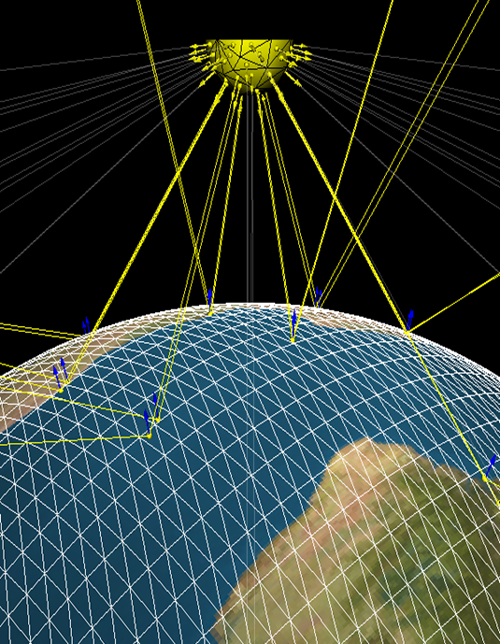Figure 6. Result of the ray-tracing example. Rays cast from the sun that missed the earth are rendered as white. Rays that intersected with the earth are rendered as yellow. The intersection points, normal vectors at the intersected earth cells, and the reflected rays can also be seen.

Conclusions

As you can see, VTK provides some little-known pearls that offer fantastic functionality.
While the above examples fall short of real-world ray-tracing applications, as one would need to account for effects like refraction and energy attenuation, the sky is the limit!

ReferencesAdamos Kyriakou is an Electrical & Computer Engineer with an MSc. in Telecommunications and a Ph.D. in Biomedical Engineering. He is currently working as a Research Associate in Computational Multiphysics at the IT’IS Foundation (ETH Zurich), where his work and research are primarily focused on computational algorithm development, high-performance computing, multi-physics simulations, big-data analysis, and medical imaging/therapy modalities.

Tags:

## 3 comments to Ray-Casting & Ray-Tracing with VTK

1.Joseph says:

The application is to Raycast a line unto a rectilinear voxelized 3D volume to determine the intersected cell IDs and the distance between crossings. I used vtkOBBTree as explained here. For the mesh, I used a mesh exported from Rhino to an stl file and read the stl file (circuitous process). Q1: Do you know of a way to create a mesh from vtkImageData or a rectilinear grid defined in a different way?
The actual app is to also compute the voxel ID enclosed by 6 cells. For example, this would be used to compute the light attenuation when crossing a voxelized 3D volume with different opacity values. I was able to extract from the cell ID the voxel ID, but the algo is very slow. Q2: Do you know of a fast method to do this, better yet, a method that produces voxelID and distance traversed through the voxel? Thank you.

1.jonatan chernyak says:

If you found an answer to Q2 I’d be very much appreciate if you share, thank you!

2.Evan says:

The answer to Q1 is to use vtkMarchingCubes or vtkFlyingEdges, which is just and optimized version of the marching cubes algorithm. If you have a 3 dimensional vtkImageData that is sdf or tsdf, it’s pretty straight forward to use those two classes.